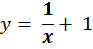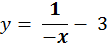### Questions − Other Graphs

 1 Draw the graph2 Draw the graph3. Draw the graph y = x3 + 27 4. Draw the graph y = −x3 − 64 5. Draw the graph y = 2x + 3 6. Draw the graph y= 2−x − 2back to:to: